Courses

# NCERT Solutions (Part- 2)- Algebraic Expressions and Identities Class 8 Notes | EduRev

## Class 8 Mathematics by VP Classes

Created by: Full Circle

## Class 8 : NCERT Solutions (Part- 2)- Algebraic Expressions and Identities Class 8 Notes | EduRev

The document NCERT Solutions (Part- 2)- Algebraic Expressions and Identities Class 8 Notes | EduRev is a part of the Class 8 Course Class 8 Mathematics by VP Classes.
All you need of Class 8 at this link: Class 8

Exercise 9.2

Question 1. Find the product of the following pairs of monomials.

(i) 4, 7p (ii) –4p, 7p (iii) –4p, 7pq
(iv) 4p3, –3p (v) 4p, 0

Solution:

(i) 4 and 7p

4 * 7p = (4 * 7) * p = 28p

(ii) –4p and 7p

–4p * 7p = {(–4) * 7} * p * p = –28p2

(iii) –4p and 7pq

–4p * 7pq = (–4 * 7) * p * qp = –28 * p2q = –28p2q

(iv) 4p3 and –3p

4p3 * (–3p) = {4 * (–3)}p3 * p = –12 * p4 = –12p4

(v) 4p and 0 ⇒ 4p * 0 = 0

Question 2. Find the areas of rectangles with the following pairs of monomials as their lengths and breadths respectively.

(p, q); (10m, 5n); (20x2, 5y2); (4*, 3x2); (3mn, 4np)

Solution:

(i) Length = p Breadth = q}             ∴ Area of the rectangle = p * q = pq

(ii) Length = 10m Breadth = 5n}     ∴ Area = 10m * 5n = 10 * 5 * m * n = 50mn

(iii) Length = 20x2 Breadth = 5y2}   ∴ Area = 20x2 * 5y= 20 * 5 * x2 * y2 = 100x2y2

(iv) Length = 4x Breadth = 3*2}       ∴ Area = 4x * 3 * x= 4 * 3 * x *x2= 12*3

(v) Length = 3mn Breadth = 4np}    ∴ Area = 3mn * 4np = (3 * 4) * m * n * n * p = 12 mn2p

Question 3. Complete the table of products.

 First monomial → Second monomial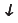2* –5y 3*2 –4*y 7*2y –9x2y2 2x–5y3x3–4xy7x2y–9x2y2 4*2…………… ……………… …–15x2y………… ……………… ……………… ………………

Solution: We have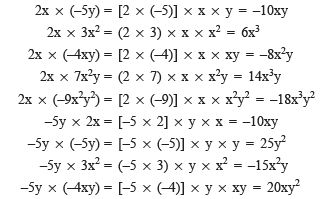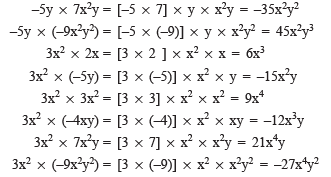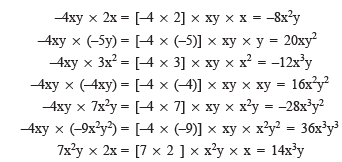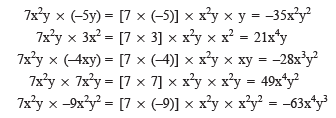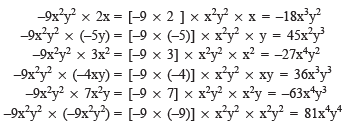First monomial → Second monomial2x -5y 3x2 -4xy 7x2y –9x2y2 2x 4x2 -10xy 6x3 -8x2y 14x3y -18x3y2 -5y -10xy 25y2 -15x2y 20xy2 -35x2y2 45x2y3 3x3 6x3 -15x2y 9x4 -12x3y 21x4y -27x4y2 -4xy -8x2y 20xy2 -12x3y 16x2y2 -28x3y2 36x3y3 7x2y 14x3y -35x2y2 21x4y -28x3y2 49x4y2 -63x4y3 -9x2y2 -18x3y2 45x2y3 -27x4y2 36x3y3 -63x4y3 81x4y4

Question 4. Obtain the volume of rectangular boxes with the following length, breadth and height respectively.

(i) 5a, 3a2, 7a4 (ii) 2p, 4q, 8r (iii) xy, 2x2y, 2xy2 (iv) a, 2b, 3c

Solution: Volume of a rectangular box = Length * Breadth * Height

(i) Length = 5a; Breadth = 3a2, Height = 7a4

∴ Volume = Length × Breadth × Height

= 5a * 3a2 * 7a4

= (5 * 3 * 7) * a * a2 * a4 = 105 * a7 = 105a7

(ii) Length = 2p, Breadth = 4q, Height = 8r

∴  Volume = Length * Breadth * Height

= 2p * 4q * 8r

= (2 * 4 * 8) * p * q * r = 64 * pqr = 64pqr

(iii) Length = xy, Breadth = 2x2y, Height = 2xy2

∴ Volume = Length* Breadth* Height

= xy* 2x2y* 2xy2

= (1* 2* 2)* xy* x2y* xy2

= 4* x4y4 = 4x4y4

(iv) Length = a, Breadth = 2b, Height = 3c

∴ Volume = Length* Breadth* Height

= a* 2b* 3c

= (1* 2* 3)* a* b* c

= 6* abc = 6abc

Question 5. Obtain the product of

(i) xy, yz, zx (ii) a, – a2, a3 (iii) 2, 4y, 8y2, 16y3 (iv) a, 2b, 3c, 6abc (v) m, – mn, mnp

Solution:

(i) xy *yz *zx = (1 *1 *1) *x *y *y *z *z *x

= 1 *(x2 *y2 *z2) = x2y2z2

(ii) a *(–a2) *a3 = [1 *(–1) *1] *a *a2 *a3

= (–1) *a6 = –a6

(iii) 2 *4y *8y2 *16y3 = (2 *4 *8 *16) *y *y2 *y3

= 1024 *y6 = 1024y6

(iv) a *2b *3c *6abc = (1 *2 *3 *6) *a *b *c *abc

= 36 *a2b2c2 = 36a2b2c2

(v) m *(–mn) *mnp = [1 *(–1) *1] *m *mn *mnp

= (–1)m3n2p = –m3n2p

Multiplying a Monomial by a Binomial

Question: Find the product

(i) 2x(3x + 5xy)   (ii) a2(2ab – 5c)

Solution:

(i) 2x(3x + 5xy) = 2x * 3x + 2x * 5xy

= (2 * 3) * x * x + (2 * 5) * x * xy

= 6 * x2 + 10 * x2y

= 6x2 + 10x2y

(ii) a2(2ab – 5c) = a2 * 2ab + a2 * (–5c)

= (1 * 2) * a2 * ab + [1 * (–5)] * a2 * c

= 2 * a3b + (–5) * a2c

= 2a3b – 5a2c

Question: Find the product: (4p2 + 5p + 7) * 3p

Solution:

(4p2 + 5p + 7) * 3p = (4p2 * 3p) + (5p * 3p) + (7 * 3p)

= [(4 * 3) * p2 * p] + [(5 * 3) * p * p] + (7 * 3) * p

= 12 * p3 + 15 * p2 + 21 * p

= 12p3 + 15p2 + 21p

Example 1. Simplify: 2x(x – 1) + 5 and find its value at x = –1

Solution:

2x(x – 1) + 5 = [2x * x] + [2x * (–1)] + 5

= [(2 * 1) * x * x] + [2 * (–1) * x] + 5

= [2 * x2] + [–2 * x] + 5

= 2x2 – 2x + 5

For x = –1, 2x2 – 2x + 5 = 2(–1)2 – 2(–1) + 5

= (2 * 1) – (–2) + 5

= 2 + 2 + 5 = 9

93 docs|16 tests

,

,

,

,

,

,

,

,

,

,

,

,

,

,

,

,

,

,

,

,

,

;